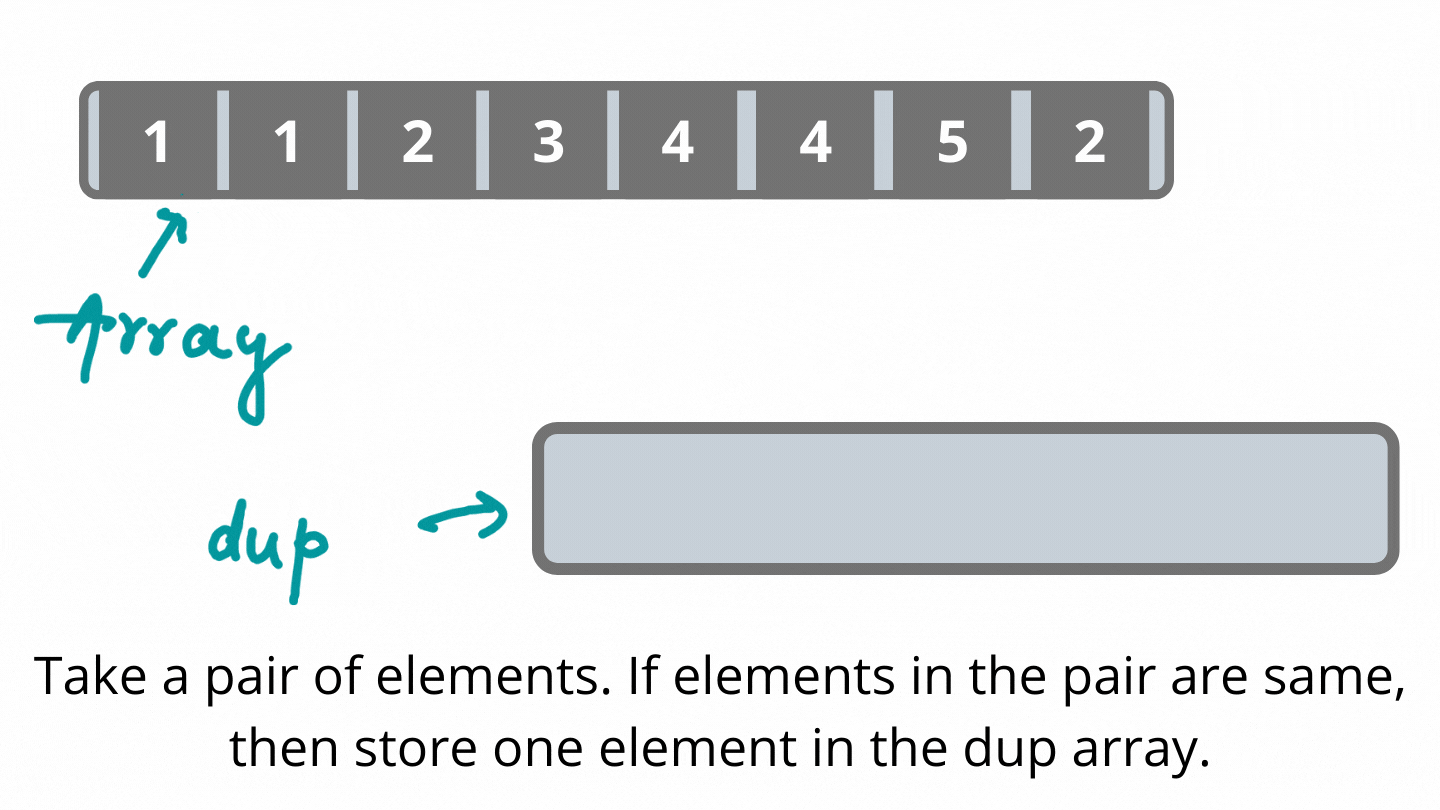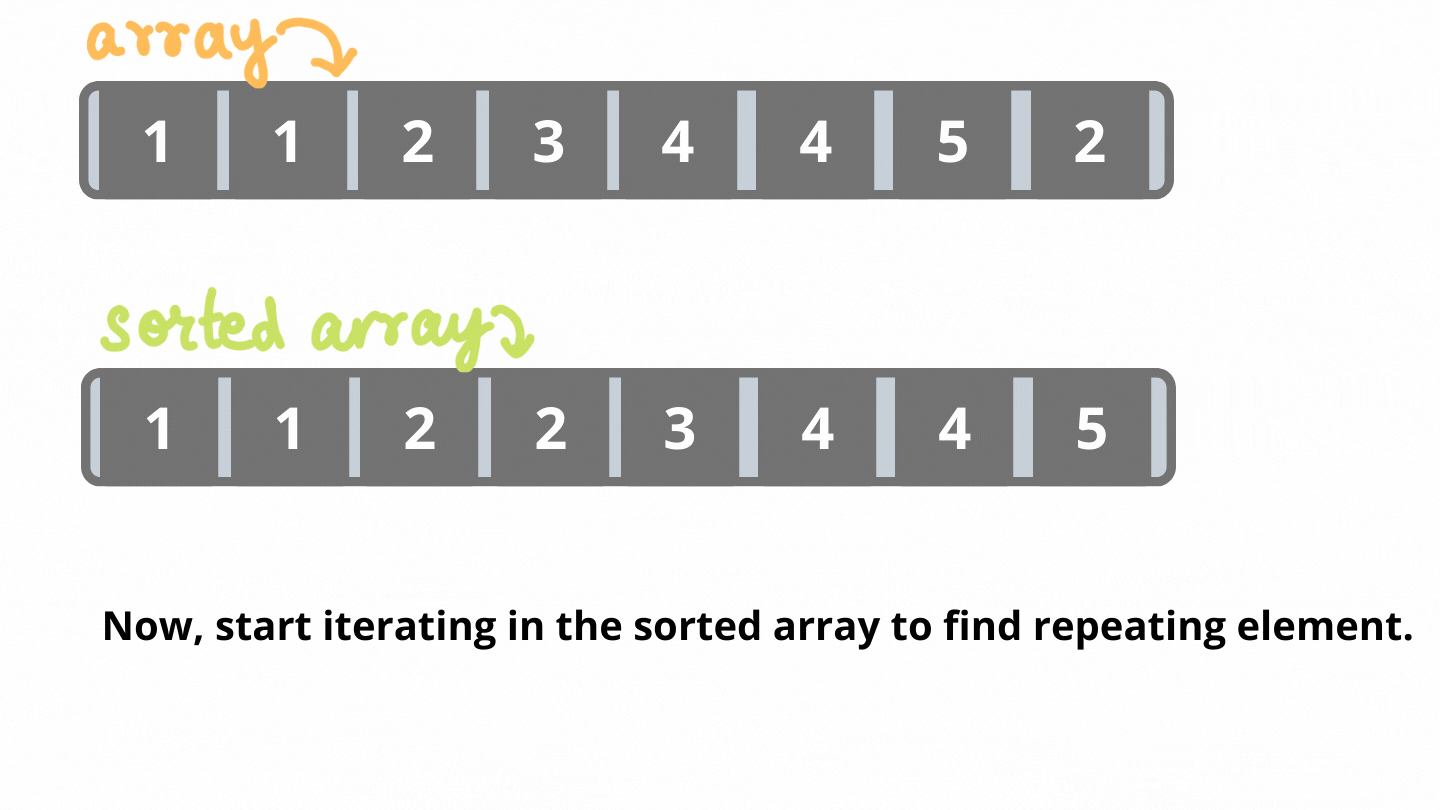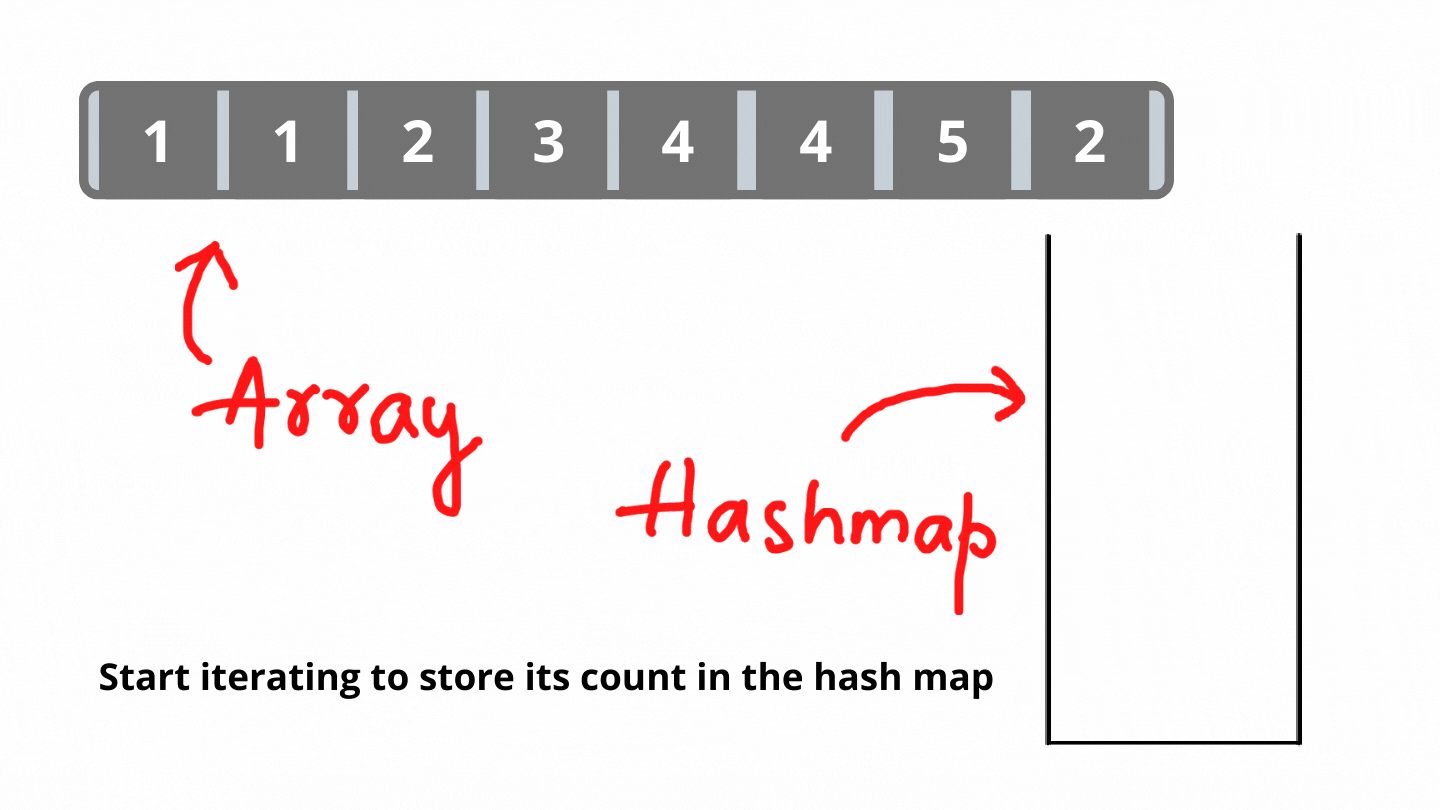# Find all repeating elements in an array

Problem Statement: Find all the repeating elements present in an array.

Examples:

```Example 1:
Input:
Arr[] = [1,1,2,3,4,4,5,2]
Output:
1,2,4
Explanation:
1,2 and 4 are the elements which are occurring more than once.

Example 2:
Input:
Arr[] = [1,1,0]
Output:
1
Explanation:
Only 1 is occurring more than once in the given array.
```

Solution 1: Brute Force

Approach:

The process is as follows:-

• Use an array to store all repeating elements. These elements are not distinct in the array. This is because for every pair of repeating elements it will store elements in the array.
• Start iterating the array. This will pick up an element for which we want to find its duplicates.
• Iterate another nested loop for finding all pairs. Pairs which have both elements are repeating elements and store them in the array created in the initial step.
• Start iterating in the array containing repeating elements.
• If the current element is not equal to the next element in the array then we print the current element. This is to ensure all unique repeating elements.

Dry Run:Code:

## C++ Code

``````#include<bits/stdc++.h>
using namespace std;
void findRepeatingElements(int arr[],int n) {
int cnt = 0;
int dup[n] ;
for(int i=0;i<n-1;i++) {
for(int j=i+1;j<n;j++) {
if(arr[i]==arr[j]) dup[cnt++] = arr[i];
}
}
cout<<"The repeating elements are: ";
for(int i=0;i<cnt;i++)
if(dup[i] != dup[i+1]) cout<<dup[i]<<" ";
}
int main() {
int arr[] = {1,1,2,3,4,4,5,2};
findRepeatingElements(arr,8);
}
``````

Output:

The repeating elements are: 1 2 4

Time Complexity: O(N2)+O(N)

Reason: We are taking one element and searching its repeating element again in the array. Also, iterating through the dup array which contains repeating elements to find unique repeating elements.

Space Complexity: O(N)

Reason: We are using an array for storing all repeating elements.

## Java Code

``````public class Main
{
static void findRepeatingElements(int arr[]) {
int cnt = 0;
int[] dup = new int[arr.length];
for(int i=0;i<arr.length-1;i++) {
for(int j=i+1;j<arr.length;j++) {
if(arr[i]==arr[j]) dup[cnt++] = arr[i];
}
}
System.out.print("The repeating elements are: ");
for(int i=0;i<cnt;i++)
if(dup[i] != dup[i+1]) System.out.print(dup[i]+" ");
}
public static void main(String[] args) {
int[] arr = {1,1,2,3,4,4,5,2};
findRepeatingElements(arr);
}
}
``````

Output:

The repeating elements are: 1 2 4

Time Complexity: O(N2)+O(N)

Reason: We are taking one element and searching its repeating element again in the array. Also, iterating through the dup array which contains repeating elements to find unique repeating elements.

Space Complexity: O(N)

Reason: We are using an array for storing all repeating elements.

Solution 2: Sorting

Approach:

The process is as follows:-

• Sort the given array.
• Start iterating in the sorted array.
• If the current element and the next element are the same, return the repeating element.

Dry Run:Code:

## C++ Code

``````#include<bits/stdc++.h>
using namespace std;

void findRepeatingElements(vector<int>& arr) {
sort(arr.begin(),arr.end());

cout<<"The repeating elements are: ";
for(int i=0;i<arr.size()-1;i++)
if(arr[i] == arr[i+1]) cout<<arr[i]<<" ";
}

int main() {
vector<int> arr = {1,1,2,3,4,4,5,2};
findRepeatingElements(arr);
return 0;
}
``````

Output:

The repeating elements are: 1 2 4

Time Complexity: O(NlogN)+O(N)

Reason: O(NlogN) for sorting. O(N) for iterating again in the array for finding a loop.

Space Complexity: O(1)

Reason: No extra spaces are used.

## Java Code

``````import java.util.Arrays;

public class Main
{
static void findRepeatingElements(int arr[]) {
Arrays.sort(arr);

System.out.print("The repeating elements are: ");
for(int i=0;i<arr.length-1;i++)
if(arr[i] == arr[i+1])
System.out.print(arr[i]+" ");
}
public static void main(String[] args) {
int[] arr = {1,1,2,3,4,4,5,2};
findRepeatingElements(arr);
}
}
``````

Output:

The repeating elements are: 1 2 4

Time Complexity: O(NlogN)+O(N)

Reason: O(NlogN) for sorting. O(N) for iterating again in the array for finding a loop.

Space Complexity: O(1)

Reason: No extra spaces are used.

Solution 3: Maps

Approach:

The process is as follows:-

• Store the elements in the hashmap with its frequency of occurrence.
• Iterate through the hashmap. If occurrence is more than 1, return the element.

Dry Run:Code:

## C++ Code

``````#include<bits/stdc++.h>
using namespace std;

void findRepeatingElements(vector<int>& arr) {
unordered_map<int,int> elementCount;
for(auto i:arr) ++elementCount[i];

cout<<"The repeating elements are: ";
for(auto i:elementCount) {
if(i.second > 1) cout<<i.first<<" ";
}
}

int main() {
vector<int> arr = {1,1,2,3,4,4,5,2};
findRepeatingElements(arr);

return 0;
}
``````

Output:

The repeating elements are: 4 1 2

Time Complexity: O(N)

Reason: Entire array is traversed to insert them in the map.

Space Complexity: O(N)

Reason: Map is used to store the count of each element.

## Java Code

``````import java.util.HashMap;
import java.util.Map;
import java.util.Map.Entry;

public class Main
{
static void findRepeatingElements(int arr[]) {
HashMap<Integer,Integer> elementCount = new HashMap<>();

for(int i:arr) {
if(elementCount.get(i) == null) elementCount.put(i,1);
else elementCount.put(i,elementCount.get(i)+1);
}
System.out.print("The repeating elements are: ");
for(Entry<Integer,Integer> entry: elementCount.entrySet()) {
if(entry.getValue()>1)
System.out.print(entry.getKey()+" ");
}

}
public static void main(String[] args) {
int[] arr = {1,1,2,3,4,4,5,2};
findRepeatingElements(arr);
}
}
``````

Output:

The repeating elements are: 1 2 4

Time Complexity: O(N)

Reason: Entire array is traversed to insert them in the map.

Space Complexity: O(N)

Reason: Map is used to store the count of each element.

Special thanks to Dewanshi Paul for contributing to this article on takeUforward. If you also wish to share your knowledge with the takeUforward fam, please check out this article